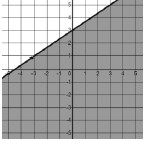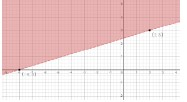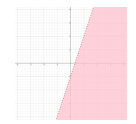# MA.912.AR.2.7Export Print
Write two-variable linear inequalities to represent relationships between quantities from a graph or a written description within a mathematical or real-world context.

### Clarifications

Clarification 1: Instruction includes the use of standard form, slope-intercept form and point-slope form and any inequality symbol can be represented.

Clarification 2: Instruction includes cases where one variable has a coefficient of zero.

General Information
Subject Area: Mathematics (B.E.S.T.)
Strand: Algebraic Reasoning
Status: State Board Approved

## Benchmark Instructional Guide

### Terms from the K-12 Glossary

• Coordinate Plane
• Linear Expression

### Vertical Alignment

Previous Benchmarks

Next Benchmarks

### Purpose and Instructional Strategies

In grade 8, students wrote two-variable linear equations. In Algebra I, students write two variable linear inequalities. In later courses, students will solve problems involving linear programming and will write two-variable quadratic inequalities.
• Instruction includes making connections to various forms of linear inequalities to show their equivalency. Students should understand and interpret when one form might be more useful than other depending on the context.
• Standard Form Can be described by the inequality $A$$x$ + $B$$y$ > $C$, where $A$, $B$ and $C$ are any rational number and any inequality symbol can be used. This form can be useful when identifying the $x$- and $y$-intercepts.
• Slope-Intercept Form Can be described by the inequality $y$ < $m$$x$ + $b$, where $m$ is the slope and $b$ is the $y$-intercept and any inequality symbol can be used. This form can be useful when identifying the slope and $y$-intercept.
• Point-Slope Form Can be described by the inequality $y$$y$$m$($x$$x$1), where ($x$1, $y$1) are a point on the boundary line and m is the slope of the line and any inequality symbol can be used. This form can be useful when the $y$-intercept is not readily apparent.
• Look for opportunities to point out the connection between linear contexts and constant rates of change.
• Problem types should include cases for vertical and horizontal lines.
• Instruction includes the connection to one-variable linear inequalities and their solutions on a number line.
• For example, provide the inequality $x$ ≥ 2 and a coordinate plane for students to determine points that satisfy the inequality. Students will determine that all points on the half-plane to the right of $x$ = 2 contain solutions.

### Common Misconceptions or Errors

• Students often choose the incorrect inequality symbol when interpreting graphs or contexts. Help these students develop the habit of using a test point to check their work. Any point can be used, but many students find it easiest to use the origin (0, 0) as it often makes mental calculation much quicker.
• When creating inequalities, students may confuse the phrase “less than.”
• For example, students interpreting the phrase “eight less than four times a number” (4$n$ − 8) could incorrectly model it as 8 − 4$n$  or 8 < 4$n$.

### Strategies to Support Tiered Instruction

• Instruction includes opportunities to use a highlighter to identify the phrases “is less than,” “is greater than,” “is less than or equal to,” and “is greater than or equal to” when writing inequalities.
• Instruction includes opportunities to identify a test point to plug into an inequality to determine which symbol should be used when writing the inequality. It is usually easiest to use the origin (0,0) as it makes mental calculations easier. If the point selected creates a true statement, their inequality is true. If it creates a false statement, their inequality is false.
• For example, given the graph below, one could use the test point (0,0) to determine whether the inequality y ≤ $\frac{\text{2}}{\text{3}}$$x$ + 3 or $y$$\frac{\text{2}}{\text{3}}$$x$ + 3 represents the graph. When plugging in (0,0) for $y$$\frac{\text{2}}{\text{3}}$$x$ + 3, it results in the inequality 0 ≥ 3 which is a false statement. When plugging in (0,0) for $y$$\frac{\text{2}}{\text{3}}$$x$ + 3, it results in the equality 0 ≤ 3 which is a true statement. Therefore, the inequality $y$$\frac{\text{2}}{\text{3}}$$x$ + 3 represents the given graph.• A carpenter makes two types of chairs: a lawn chair and a living room chair. It takes her 3 hours to make a lawn chair and 5 hours to make a living room chair.
• Part A. If the carpenter works a maximum of 55 hours per week, write a two-variable linear inequality to describe the number of possible chairs of each type she can make in a week.
• Part B. What is one possible combination of lawn chair and living room chair that the carpenter can make in one week?
• Part C. What is one possible combination of lawn chair and living room chair that the carpenter could make in one month?

• The graph of the solution set to a linear inequality is shown below.• Part A. Create a linear inequality that corresponds to this graph.
• Part B. Create a real-world situation that could be described by the graph and the inequality created in Part A.

### Instructional Items

Instructional Item 1
• The graph of the solution set to a linear inequality is shown below. Create a linear inequality that corresponds to this graph.*The strategies, tasks and items included in the B1G-M are examples and should not be considered comprehensive.

## Related Courses

This benchmark is part of these courses.
1200310: Algebra 1 (Specifically in versions: 2014 - 2015, 2015 - 2022, 2022 and beyond (current))
1200320: Algebra 1 Honors (Specifically in versions: 2014 - 2015, 2015 - 2022, 2022 and beyond (current))
1200370: Algebra 1-A (Specifically in versions: 2014 - 2015, 2015 - 2022, 2022 and beyond (current))
1200400: Foundational Skills in Mathematics 9-12 (Specifically in versions: 2014 - 2015, 2015 - 2022, 2022 and beyond (current))
7912080: Access Algebra 1A (Specifically in versions: 2014 - 2015, 2015 - 2018, 2018 - 2019, 2019 - 2022, 2022 and beyond (current))
1200315: Algebra 1 for Credit Recovery (Specifically in versions: 2014 - 2015, 2015 - 2022, 2022 and beyond (current))
1200375: Algebra 1-A for Credit Recovery (Specifically in versions: 2014 - 2015, 2015 - 2022, 2022 and beyond (current))
7912075: Access Algebra 1 (Specifically in versions: 2014 - 2015, 2015 - 2018, 2018 - 2019, 2019 - 2022, 2022 and beyond (current))

## Related Access Points

Alternate version of this benchmark for students with significant cognitive disabilities.
MA.912.AR.2.AP.7: Select a two-variable linear inequality to represent relationships between quantities from a graph.

## Related Resources

Vetted resources educators can use to teach the concepts and skills in this benchmark.

## Student Resources

Vetted resources students can use to learn the concepts and skills in this benchmark.

## Parent Resources

Vetted resources caregivers can use to help students learn the concepts and skills in this benchmark.